# Nonlinear Statics

### Geometrically nonlinear behavior

This module part allows for the geometric nonlinear analysis of models. There, large displacements with small strains (i.e. linear elastic material behavior) are assumed. Beside an automatic load step control different nonlinear solvers are available (like Newton-Raphson, Modified Newton-Raphson, Quasi Newton BFGS, Secant Newton, Line search, Arc length method).
The nonlinear characteristic may be represented by xy-plots, where the load increments can be chosen automatically or manually.

### Material nonlinearities

Analysis of nonlinear material behaviour of models with small strains under isotropic conditions:

• Nonlinear elasticity (of Cauchy type)
• Plasticity (von Mises, Tresca, Drucker-Prager, Mohr-Coulomb)
• Visco-plasticity of power-law type for von Mises yield criterion.
• Creep with
• nonlinear elasticity or
• plasticity

The material can be defined temperature-dependent for Young's modulus, yield stress, and the stress-strain curves. A time-dependent characteristic is present for creep calculations in addition. Hardening in plasticity can be defined isotropic or kinematic (or mixed).

For the use of shell elements with material nonlinearities, an element family of elements with a 3-dimensional shell formulation is available, which is applicable for linear analyses, too. This element family (triangles and quadrangles with linear and quadratic shape functions) has been designed for nonlinear analysis with already existing shell models.

For the modeling of gaskets a family of gasket elements is available. These elements are used to define the nonlinear behavior in a preferential direction by a measured force-displacement curve of the real gasket.

An incremental and iterative solver strategy is based on Newton-Raphson, Modified Newton-Raphson, and Thomas method. An automatic load step control allows for an optional specification of initial load step and total applied load (or time). The material laws may be defined either in tabular form or as user-written subroutine (Fortran or C).

Applications using inertia relief can also take nonlinear material behavior into account.

Structural behavior can be influenced by any pretreatment(like casting, rolling). The resulting internal strains can be used as initial conditions (without displacements).

User defined material laws can be used. User subroutines allow the incorporation of own material laws. The subroutine does the necessary calculation of stresses and strains together with the tangent matrix associated with the material law.

### Combination of material and geometrical nonlinearities

Analyses with material nonlinearities can take into account the geometrical nonlinear effects, too. There, also follower loads like pressure loads, temperature loads, and inertia loads can be taken into account.

### Generals

In case of contact definitions the nonlinear analysis takes them into account automatically performing a nonlinear contact analysis.

Extrapolation of stresses for linear and nonlinear elements is fully provided. For linear elements, stresses are calculated at the stress points and either assigned or extrapolated to the element corner nodes. For nonlinear elements, stresses are calculated at the integration points and either assigned to the element nodes or extrapolated to the element corner nodes. If stresses are not assigned to the element midside nodes, these stresses will be calculated through linear interpolation from the element corner nodes. In plasticity analysis, stresses at the element nodes are showing stresses at the integration points or they are showing extrapolated stresses which do not lie on the yield surface any more. In case of subsequent generation of nodal point stresses in post-processing, the original element stress results can be seriously modified.

Initial states like for rotating structures can be taken into account in nonlinear analyses.

The results of a nonlinear analysis may be used for subsequent analysis like a dynamic mode analysis.

In many cases, the major part of the model is linear. This is an ideal prerequisite to apply substructure technique, where the linear parts are put in subcomponents and all nonlinear parts are put in the top component. This procedure will lead to a significant reduction in run time.

If many loading cycles have to be calculated for realistic models (e.g. with creep in engine models), then a recommendable strategy is to use submodelling to reduce the the model to the area of interest. The results of the global analysis are mapped to a submodel. Then, the nonlinear analysis will be performed for the submodel. Run times are reduced dependent on the size of the submodel and may allow for time consuming nonlinear analysis.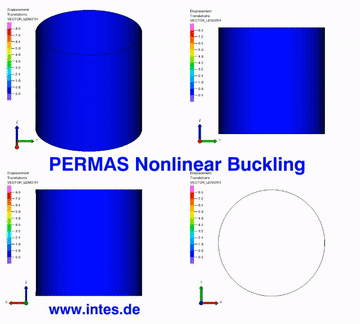Nonlinear buckling of a composite cylinder shell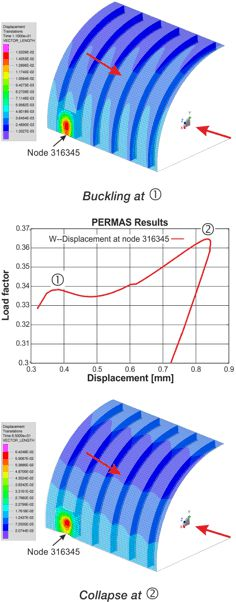Plastic buckling of a reinforced cylinder shellPlastic failure of bolt thread under tension.

### Hyperelastic Material

• A complete set of volume elements is available for large strain analysis. The selection is performed automatically by assigning hyperelastic material properties.
• Several strain potential types available:
• Polynomial
• Reduced Polynomial
• Neo Hooke
• Mooney-Rivlin
• Yeoh
• Ogden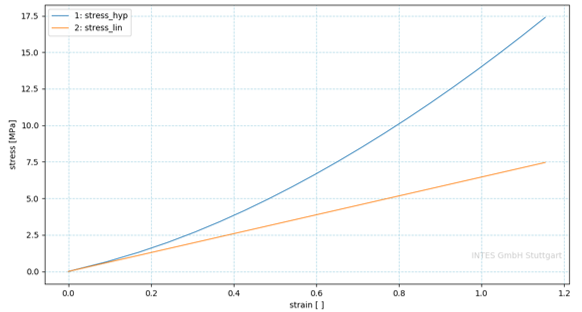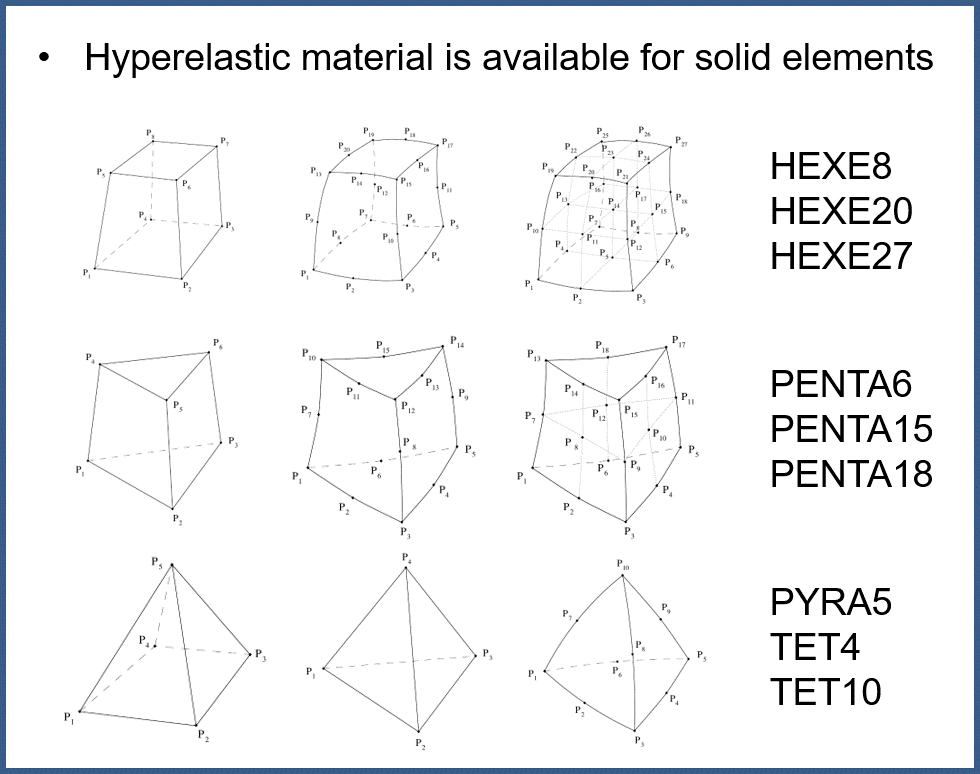Linear and hyperelastic material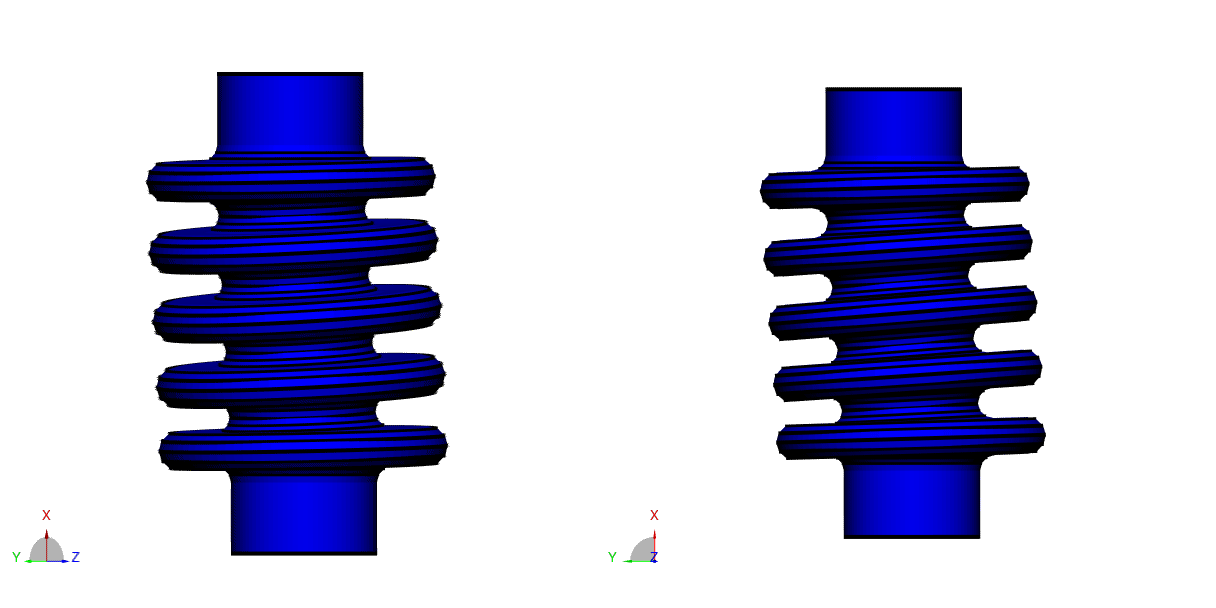Simulation of a bellow with hyperelastic material.